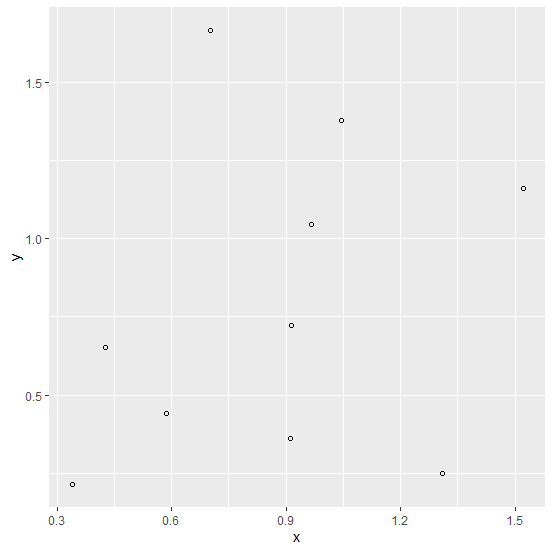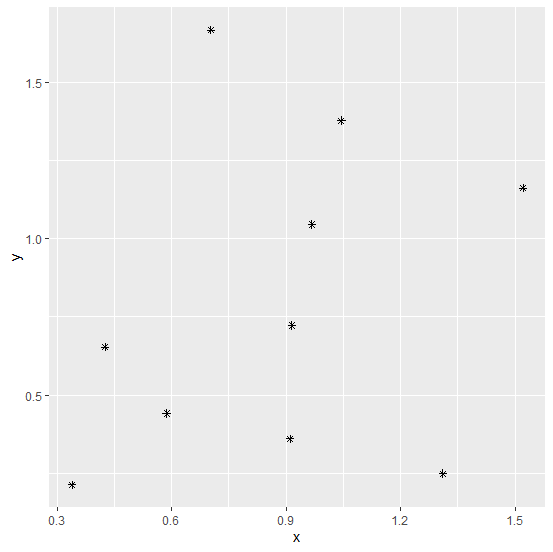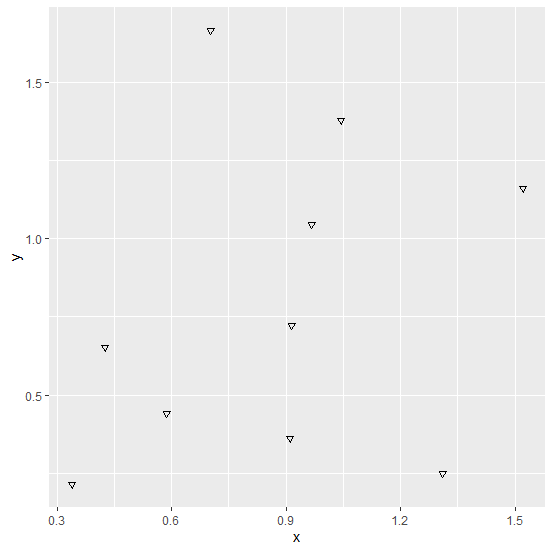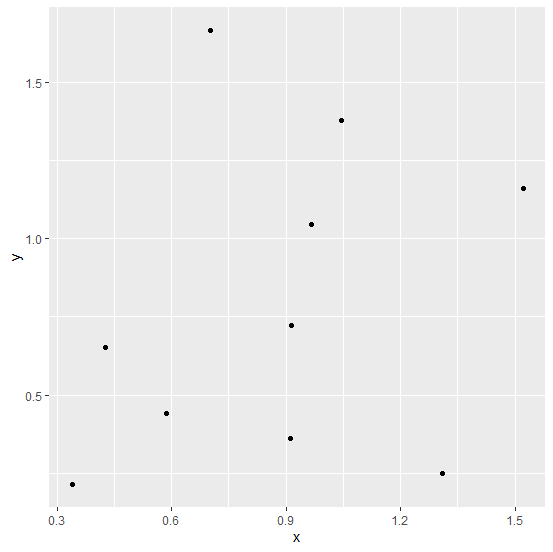# What are the different types of point available in geom_point of ggplot2 package in R?

We can create a point chart using ggplot2 package but that point not necessarily to be in circular shape, we have twenty-five shape options for those points in ggplot2. While creating a point chart using ggplot2, we can use shape argument inside geom_point to see the difference among these twenty-five shapes.

## Example

Consider the below data frame:

Live Demo

> set.seed(1957)
> x<-rnorm(10,1,0.38)
> y<-rnorm(10,1,0.67)
> df<-data.frame(x,y)
> df

## Output

       x       y
1 0.7028704 1.6664500
2 0.9672393 1.0456639
3 1.3102736 0.2495795
4 0.3389941 0.2141513
5 0.5867095 0.4417377
6 0.4257543 0.6533757
7 0.9106756 0.3611954
8 1.0444729 1.3770588
9 0.9151447 0.7225429
10 1.5207510 1.1613454

Loading ggplot2 package and creating a point chart with shape = 1:

## Example

> library(ggplot2)
> ggplot(df,aes(x,y))+geom_point(shape=1)

## Output:Creating a point chart with shape = 8:

## Example

> ggplot(df,aes(x,y))+geom_point(shape=8)

## Output:Creating a point chart with shape = 25:

## Example

> ggplot(df,aes(x,y))+geom_point(shape=25)

## Output:Creating a point chart with shape = 16:

## Example

> ggplot(df,aes(x,y))+geom_point(shape=16)

## Output: